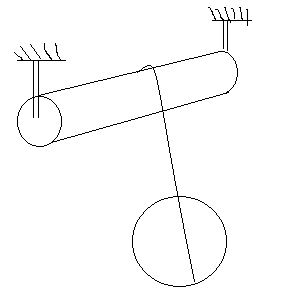# Aditya's challenges in Mechanics 8The arrangement shown above consists of a solid cylinder and a solid sphere, each of mass $1\text{ kg}$, on which a light thread is wound. Find the tension in the thread in the process of motion.

The answer is of the form $\frac{A}{B}$ for coprime positive integers $A$ and $B$. Find $A+B$.

Details and Assumptions:

• The radii of of the cylinder and sphere are equal and are equal to $1m$

• Take acceleration due to gravity as $g=10\text{ ms}^{-1}$.

• The friction in the axle of the upper cylinder is assumed to be absent.

• There is no slipping anywhere.

×